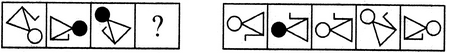# Non Verbal Reasoning - Analogy - Discussion

### Discussion :: Analogy - Section 1 (Q.No.12)

Each of the following questions consists of two sets of figures. Figures A, B, C and D constitute the Problem Set while figures 1, 2, 3, 4 and 5 constitute the Answer Set. There is a definite relationship between figures A and B. Establish a similar relationship between figures C and D by selecting a suitable figure from the Answer Set that would replace the question mark (?) in fig. (D).

12.

Select a suitable figure from the Answer Figures that would replace the question mark (?).(A)     (B)      (C)     (D)                  (1)      (2)      (3)      (4)      (5)

 [A]. 1 [B]. 2 [C]. 3 [D]. 4 [E]. 5

Explanation:

The figure rotates 45oACW; the circle changes colour (turns black if initially white and vice-versa). The 'L'-shaped element shifts to the other side of the main figure.

 Anu said: (Mar 13, 2012) From fig. A to B its clear that it is turning 45 Degree ACW. But after that its turning through 135 Degrees. If so what wil be the answer?.